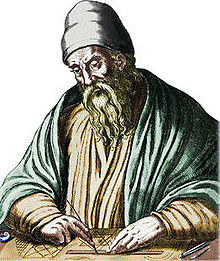# Misunderstanding Euclid's argumentA common flawed presentation of Euclid's proof of the infinitude of primes is as follows:

Assume there are only a finite number of primes $p_1,p_2,\ldots,p_n$. Let $N$ be the product of all of those primes, add to it $1$ and you get a new prime number since it isn't divisible by any of the primes we listed at first. Contradiction! $\Rightarrow\Leftarrow$ Therefore, there is an infinite number of primes.

Let $q_1, q_2, q_3, \ldots$ be the list of all primes (in ascending order). Your mission is to find the smallest value of $q_1 q_2 q_3 \ldots q_n + 1$ that is not a prime.

×